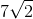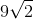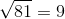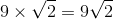# PSAT Math : How to find the length of the diagonal of a square

## Example Questions

### Example Question #1 : Squares

If the area of a square isunits squared, what is the length of its diagonal?unitsunitsunits

Not Enough Informationunitsunits

Explanation:

The diagonal of a square creates two special 45-45-90 triangles, meaning that the diagonal of a square is just the length of one side of the square multiplied by the square root of 2.

In this problem, you can figure out the length of one side of the square by finding the square root of the area (which is equal to a side length), then multiplying that number by the square root of 2.